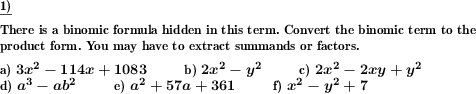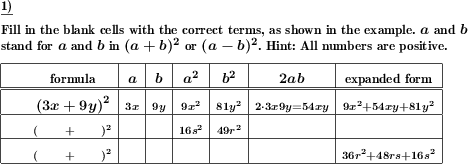Custom math worksheets at your fingertips# Details for problem "Simplify term with binomic formula, combine like terms"

Quickname: 1444

Elementary School, Primary School, Junior High School, Middle School, High School.

## Summary

Simplify a term which contains a binomic formula

## Example## Description

Use your knowledge about the parentheses and expanded form of binomic formulas to quickly expand a term. Then combine like terms to obtain a shorter term.

A complex term ist given. The task is to expand all products and simplify the term by combining like terms. Part of the term is an easily recognizable binomic formula. Expand the binomic formular, perform other operations and expansions as required and combine like terms.

The summands of the formula's factors will be either a variable and a literal number or both of them will be variables. In no case, both summands will be numbers, which means the expanded term will always contain variables and it does not simplify to a single literal number.

The number of problems is selectable. For the types of formulas that will appear it can be specified that only some of the three binomic formulas will be used. The fact that a binomic formular appears in the term can be selected to be mentioned or omitted.

The type of term the binomic formula will be an operand of can be specified.

Variables that appear will always be named a and b or x and y.

Download free printable worksheets for this math problem here. The worksheet contains the problems only, the solution sheet includes the answers. Just click on the respective link.

•Worksheet 1Solution sheet with answers
•Worksheet 2Solution sheet with answers
•Worksheet 3Solution sheet with answers

If you can not see the solution sheets for download, they may be filtered out by an ad blocker that you may have installed. If this is the case, please allow ads for this page and reload the page. The solution sheets will then reappear.

• Do these sample worksheets do not really fit?
• Do you need more math worksheets, with a different level of difficulty?
• Would you like to combine different problems on a worksheet and adjust them to your needs?
• As a teacher, you can put together your own worksheets using the automatically generated math problems provided.
With a free initial credit, you can start creating your own math worksheets in a few minutes.

You can try it for free! Register here, to create custom worksheets now!

## Customization options for this problem

Parameter
Possible values
Number of problems
1, 2, 3, 4, 5, 6, 7, 8, 9, 10
Binomic formula type
(a+b)^2, (a-b)^2, (a+b)(a-b), (a+b)^2 & (a-b)^2, all
Part of
product,sum,difference, product, sum, sum,difference
Second term
variable,number, variable, number
Mention binomic formulas
Yes, No

## Similar problems

Remark
Description
Just expand and simplify
Apply binomic formula expansion rules to simplify term.
Opposite problem
A binomic formula is hidden in a term. Factor out the binomic formular or extract the summand.

## dw-Math worksheet templates that do contain this problem

Name
Title
Description
Use the binomic formulas to simplify and expand terms.

## Other types of problems that appear on worksheets with this problem:

Relevance
Name
Description
Quickname
Example
****
Expand and simplify binomic formula, advanced
Apply binomic formula expansion rules to simplify term. Advanced version mit multiples of variables as summands.****
Extract binomic formula from term
A binomic formula is hidden in a term. Factor out the binomic formular or extract the summand.****
Examine the elements of binomic formulas
Elements of a binomic formula and their expanded form are grouped in a table. Fill the empty spaces.